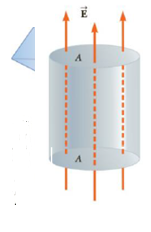Chapter 15, Problem 49P

Chapter
Section
Textbook Problem

Figure P15.49 shows a closed cylinder with cross-sectional area A = 2.00 m2. The constant electric field E → has magnitude 3.50 × 103 N/C and is directed vertically upward, perpendicular to the cylinder's top and bottom surfaces so that no field lines paw through the curved surface. Calculate the electric flux through the cylinder's (a) lop and (b) bottom surface, (c) Determine the amount of charge inside the cylinder.Figure P15.49

(a)

To determine
The electric flux through the top surface.

Explanation

Given info: The electric field (E) is 3.5×103N/C . The area of the surface (A) is 2.00m2 .

The angle between the electric field and area vector ( θ ) is 0ο .

Formula to calculate the electric flux is,

ϕE=EAcosθ

Substitute 3.5×103N/C for E, 2.00m2 for A and 0ο for θ

(b)

To determine
The electric flux through the bottom surface.

(c)

To determine
The charge inside the cylinder.

Still sussing out bartleby?

Check out a sample textbook solution.

See a sample solution

The Solution to Your Study Problems

Bartleby provides explanations to thousands of textbook problems written by our experts, many with advanced degrees!

Get Started